Search by Topic

Resources tagged with Interactivities similar to Sort the Street:

Filter by: Content type:
Age range:
Challenge level:

There are 141 results

Broad Topics > Information and Communications Technology > Interactivities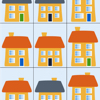Sort the Street

Age 5 to 7 Challenge Level:

Sort the houses in my street into different groups. Can you do it in any other ways?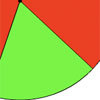Turning

Age 5 to 7 Challenge Level:

Use your mouse to move the red and green parts of this disc. Can you make images which show the turnings described?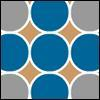Counters

Age 7 to 11 Challenge Level:

Hover your mouse over the counters to see which ones will be removed. Click to remove them. The winner is the last one to remove a counter. How you can make sure you win?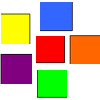Coloured Squares

Age 5 to 7 Challenge Level:

Use the clues to colour each square.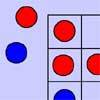Red Even

Age 7 to 11 Challenge Level:

You have 4 red and 5 blue counters. How many ways can they be placed on a 3 by 3 grid so that all the rows columns and diagonals have an even number of red counters?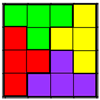Tetrafit

Age 7 to 11 Challenge Level:

A tetromino is made up of four squares joined edge to edge. Can this tetromino, together with 15 copies of itself, be used to cover an eight by eight chessboard?More Transformations on a Pegboard

Age 7 to 11 Challenge Level:

Use the interactivity to find all the different right-angled triangles you can make by just moving one corner of the starting triangle.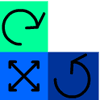Tessellate the Triominoes

Age 5 to 7 Challenge Level:

What happens when you try and fit the triomino pieces into these two grids?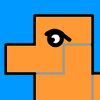Cover the Camel

Age 5 to 7 Challenge Level:

Can you cover the camel with these pieces?Four Triangles Puzzle

Age 5 to 11 Challenge Level:

Cut four triangles from a square as shown in the picture. How many different shapes can you make by fitting the four triangles back together?Seven Flipped

Age 7 to 11 Challenge Level:

Investigate the smallest number of moves it takes to turn these mats upside-down if you can only turn exactly three at a time.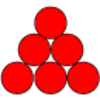Find the Difference

Age 5 to 7 Challenge Level:

Place the numbers 1 to 6 in the circles so that each number is the difference between the two numbers just below it.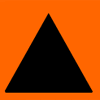Sorting Symmetries

Age 7 to 11 Challenge Level:

Find out how we can describe the "symmetries" of this triangle and investigate some combinations of rotating and flipping it.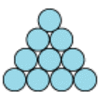Difference

Age 7 to 11 Challenge Level:

Place the numbers 1 to 10 in the circles so that each number is the difference between the two numbers just below it.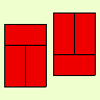Fault-free Rectangles

Age 7 to 11 Challenge Level:

Find out what a "fault-free" rectangle is and try to make some of your own.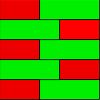Combining Cuisenaire

Age 7 to 11 Challenge Level:

Can you find all the different ways of lining up these Cuisenaire rods?Making Trains

Age 5 to 7 Challenge Level:

Can you make a train the same length as Laura's but using three differently coloured rods? Is there only one way of doing it?Same Length Trains

Age 5 to 7 Challenge Level:

How many trains can you make which are the same length as Matt's, using rods that are identical?Nine-pin Triangles

Age 7 to 11 Challenge Level:

How many different triangles can you make on a circular pegboard that has nine pegs?Triangles All Around

Age 7 to 11 Challenge Level:

Can you find all the different triangles on these peg boards, and find their angles?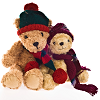Teddy Town

Age 5 to 14 Challenge Level:

There are nine teddies in Teddy Town - three red, three blue and three yellow. There are also nine houses, three of each colour. Can you put them on the map of Teddy Town according to the rules?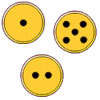Fair Exchange

Age 5 to 7 Challenge Level:

In your bank, you have three types of coins. The number of spots shows how much they are worth. Can you choose coins to exchange with the groups given to make the same total?Two and One

Age 5 to 7 Challenge Level:

Terry and Ali are playing a game with three balls. Is it fair that Terry wins when the middle ball is red?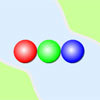Three Ball Line Up

Age 5 to 7 Challenge Level:

Use the interactivity to help get a feel for this problem and to find out all the possible ways the balls could land.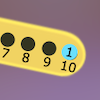Are You Well Balanced?

Age 5 to 7 Challenge Level:

Can you work out how to balance this equaliser? You can put more than one weight on a hook.Code Breaker

Age 7 to 11 Challenge Level:

This problem is based on a code using two different prime numbers less than 10. You'll need to multiply them together and shift the alphabet forwards by the result. Can you decipher the code?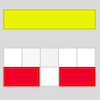Cuisenaire Counting

Age 5 to 7 Challenge Level:

Here are some rods that are different colours. How could I make a dark green rod using yellow and white rods?Arrangements

Age 7 to 11 Challenge Level:

Is it possible to place 2 counters on the 3 by 3 grid so that there is an even number of counters in every row and every column? How about if you have 3 counters or 4 counters or....?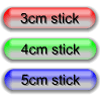Triangle Edges

Age 5 to 7 Challenge Level:

How many triangles can you make using sticks that are 3cm, 4cm and 5cm long?Growing Garlic

Age 5 to 7 Challenge Level:

Ben and his mum are planting garlic. Use the interactivity to help you find out how many cloves of garlic they might have had.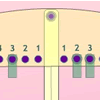Getting the Balance

Age 5 to 7 Challenge Level:

If you hang two weights on one side of this balance, in how many different ways can you hang three weights on the other side for it to be balanced?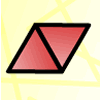Triangle Animals

Age 5 to 7 Challenge Level:

How many different ways can you find to join three equilateral triangles together? Can you convince us that you have found them all?Matching Triangles

Age 5 to 7 Challenge Level:

Can you sort these triangles into three different families and explain how you did it?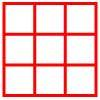Age 5 to 11 Challenge Level:

Place six toy ladybirds into the box so that there are two ladybirds in every column and every row.A Square of Numbers

Age 7 to 11 Challenge Level:

Can you put the numbers 1 to 8 into the circles so that the four calculations are correct?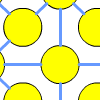One to Fifteen

Age 7 to 11 Challenge Level:

Can you put the numbers from 1 to 15 on the circles so that no consecutive numbers lie anywhere along a continuous straight line?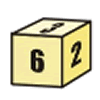First Connect Three for Two

Age 7 to 11 Challenge Level:

First Connect Three game for an adult and child. Use the dice numbers and either addition or subtraction to get three numbers in a straight line.Multiples Grid

Age 7 to 11 Challenge Level:

What do the numbers shaded in blue on this hundred square have in common? What do you notice about the pink numbers? How about the shaded numbers in the other squares?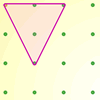Inside Triangles

Age 5 to 7 Challenge Level:

How many different triangles can you draw on the dotty grid which each have one dot in the middle?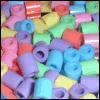Age 7 to 11 Challenge Level:

Three beads are threaded on a circular wire and are coloured either red or blue. Can you find all four different combinations?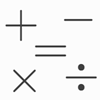Which Symbol?

Age 7 to 11 Challenge Level:

Choose a symbol to put into the number sentence.Junior Frogs

Age 5 to 11 Challenge Level:

Have a go at this well-known challenge. Can you swap the frogs and toads in as few slides and jumps as possible?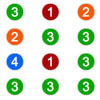Winning the Lottery

Age 7 to 11 Challenge Level:

Try out the lottery that is played in a far-away land. What is the chance of winning?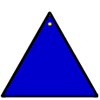Posting Triangles

Age 5 to 7 Challenge Level:

If you can post the triangle with either the blue or yellow colour face up, how many ways can it be posted altogether?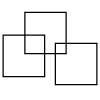Three Squares

Age 5 to 11 Challenge Level:

What is the greatest number of squares you can make by overlapping three squares?Domino Numbers

Age 7 to 11 Challenge Level:

Can you see why 2 by 2 could be 5? Can you predict what 2 by 10 will be?Coded Hundred Square

Age 7 to 11 Challenge Level:

This 100 square jigsaw is written in code. It starts with 1 and ends with 100. Can you build it up?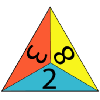One Big Triangle

Age 5 to 7 Challenge Level:

Make one big triangle so the numbers that touch on the small triangles add to 10.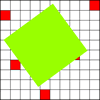How Random!

Age 7 to 11 Challenge Level:

Explore this interactivity and see if you can work out what it does. Could you use it to estimate the area of a shape?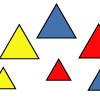Sorting Shapes

Age 5 to 7 Challenge Level:

This activity challenges you to make collections of shapes. Can you give your collection a name?